# The perimeter of problems

#### Number of problems found: 448

• Dimensions 3A perimeter of a rectangular field is 96 meters. The length is four meters less than three times the width. Find the length and width (its dimensions).
• Matilda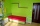Matilda measures the perimeter of her bedroom to determine how many feet of border she needs to go around the tops of the walls. Two walls are each 96 inches long, and two walls are each 3 yards long. She pays \$30 for border that costs \$1 per foot. What a
• Perimeter - rectangleFind the perimeter of the rectangle. A rectangle with a base of (3 5/6) m and a height of (2 3/7) m Its perimeter is x= meters. (Use fraction in your answer. )
• Find a 2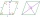Find a length of the diagonal AC of the rhombus ABCD if its perimeter P = 112 dm and the second diagonal BD has a length of 36 dm.
• One of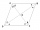One of the internal angles of the rhombus is 120° and the shorter diagonal is 3.4 meters long. Find the perimeter of the rhombus.
• A perimeter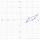A perimeter of a parallelogram is 2.8 meters. The length of one of its sides is equal to one-seventh of the entire perimeter. Find lengths of the sides of the parallelogram.
• A rectangular 4A rectangular garden has a length of 57 m and a width of 42 m. Calculate of how many m2 will decrease the area of a garden, if the ornamental fence with a width of 60 cm will be planted inside its perimeter.
• Perimeter to area 2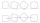Find the area of a square whose perimeter is 100 cm with solve.
• Length 9Length of a rectangle is 8 m less than twice of breadth. If the perimeter of the rectangle is 50. find its length and breadth.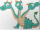Three sides of a quadrilateral are equal to the foreside which is 16 cm long. What is the length of the one with equal sides if the perimeter is 58 cm?
• Dimensions of rectangleFind the dimensions of a rectangle given that its perimeter is 110 cm and its length is 1 cm more than twice its width.
• Perimeter of a triangle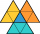If the perimeter of a triangle is 6 2/3 cm and the lengths of two of its sides are 2 1/2 cm and 3 1/3 cm, find the length if the third side
• The perimeter 6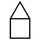The perimeter of quadrilateral is 442.95 cm. If the three known sides measure 84.56 cm,132.77 cm, and 82.75 cm, how long is the fourth side?
• Polygon - area coordinatesFind the perimeter and the area of the polygon with the given vertices. T (2,7), U (2,9), V (5,9), W (5,7)
• What is 8What is the perimeter of a rectangle with side lengths of ¾ inch and ⅔?
• Area and perimeter 2Find the area and the perimeter of a rectangle of length 45 1/2 cm and breadth 16 2/3 cm.
• The length 4The length of a rectangular room measures twice a number x increased by 5. The width measures a number x increased by 3. What is the perimeter of the room?
• The homeowner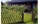The homeowner plans to build a fence along the sides and back of the property. How many feet of fencing are required to build the fence? (1 metre = 3.2808399 feet)Lots: 14w x 36.6d (m)
• Centre of the hypotenuseFor the interior angles of the triangle ABC, alpha beta and gamma are in a ratio of 1: 2: 3. The longest side of the AB triangle is 30 cm long. Calculate the perimeter of the triangle CBS if S is the center of the side AB.
• Diamond PQOR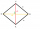In the diamond PQOR, the diagonal RQ is 4 cm long and the angle RPQ is 60°. What is the circumference of this diamond?

Do you have an exciting math question or word problem that you can't solve? Ask a question or post a math problem, and we can try to solve it.

We will send a solution to your e-mail address. Solved examples are also published here. Please enter the e-mail correctly and check whether you don't have a full mailbox.

Please do not submit problems from current active competitions such as Mathematical Olympiad, correspondence seminars etc...